# Noetherian implies every element in minimal prime is zero divisor

This article defines a result where the base ring (or one or more of the rings involved) is Noetherian
View more results involving Noetherianness or Read a survey article on applying Noetherianness

## Statement

In a Noetherian ring, any element that lies in a minimal prime ideal must be a zero divisor.

## Converse

The converse statement is true when the ring is reduced. Further information: reduced Noetherian implies zero divisor in minimal prime

## Generalizations

The assumption that the ring be Noetherian can be weakened to the assumption that every prime contains a minimal prime, and such that there are only finitely many minimal primes.

## Facts used

1. Noetherian ring has finitely many minimal primes and every prime contains a minimal prime

## Proof

Given: A Noetherian ring$R$

To prove: If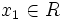$x_1 \in R$ lies in a minimal prime ideal of$R$, then$x$ is a zero divisor.

Proof: Since$R$ is a Noetherian ring, it has only finitely many minimal primes, say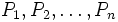$P_1, P_2, \ldots, P_n$ (and without loss of generality,$x_1 \in P_1$. Moreover, any prime ideal contains a minimal prime ideal, so the nilradical of$R$ equals the intersection of the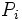$P_i$s. In particular, the product of the$P_i$s lies inside the nilradical of$R$.

Let us next consider the product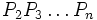$P_2 P_3 \ldots P_n$. If this product is contained in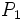$P_1$, then, one of the$P_j$s is contained in$P_1$, contradicting the assumption of minimality. Thus,$P_2P_3\ldots P_n$ is not contained in$P_1$, so we can find elements$x_j \in P_j, 2 \le j \le n$ such that$x_2x_3 \ldots x_n \notin P_1$.

Now consider the product$x_1x_2x_3 \ldots x_n$. This product lies in the nilradical, hence some power of it is zero. Thus, there exists a$j$ such that:$x_1^j(x_2x_3 \ldots x_n)^j = 0$

However, the expression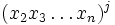$(x_2 x_3 \ldots x_n)^j$ cannot be zero because$x_2x_3 \ldots x_n \notin P_1$, and therefore, is not nilpotent.

Thus,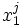$x_1^j$ is a zero divisor, and hence,$x_1$ is a zero divisor (that's because the set of nonzerodivisors is a multiplicatively closed subset, so if$x_1$ were not a zero divisor,$x_1^j$ would also not be a zero divisor).